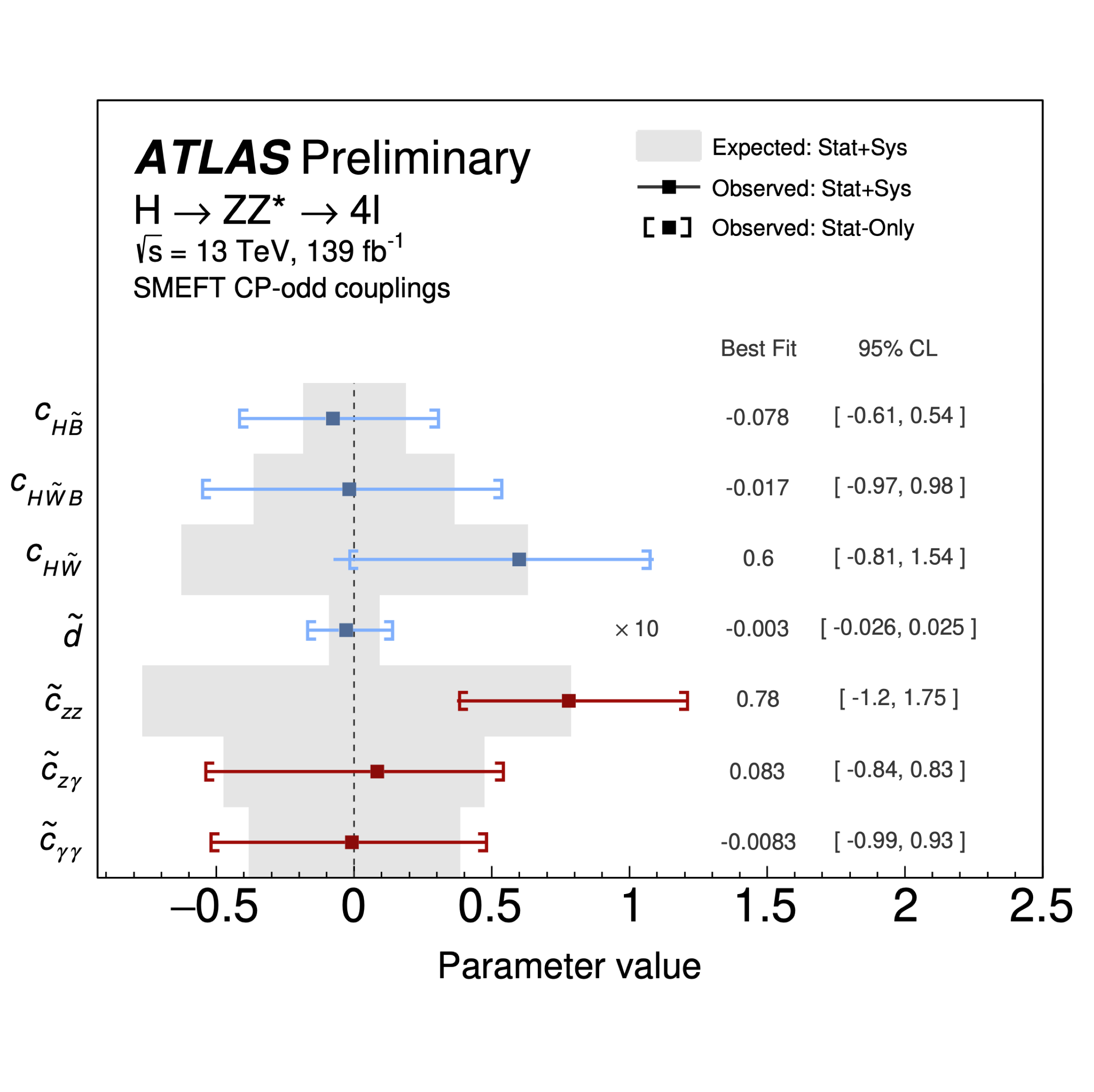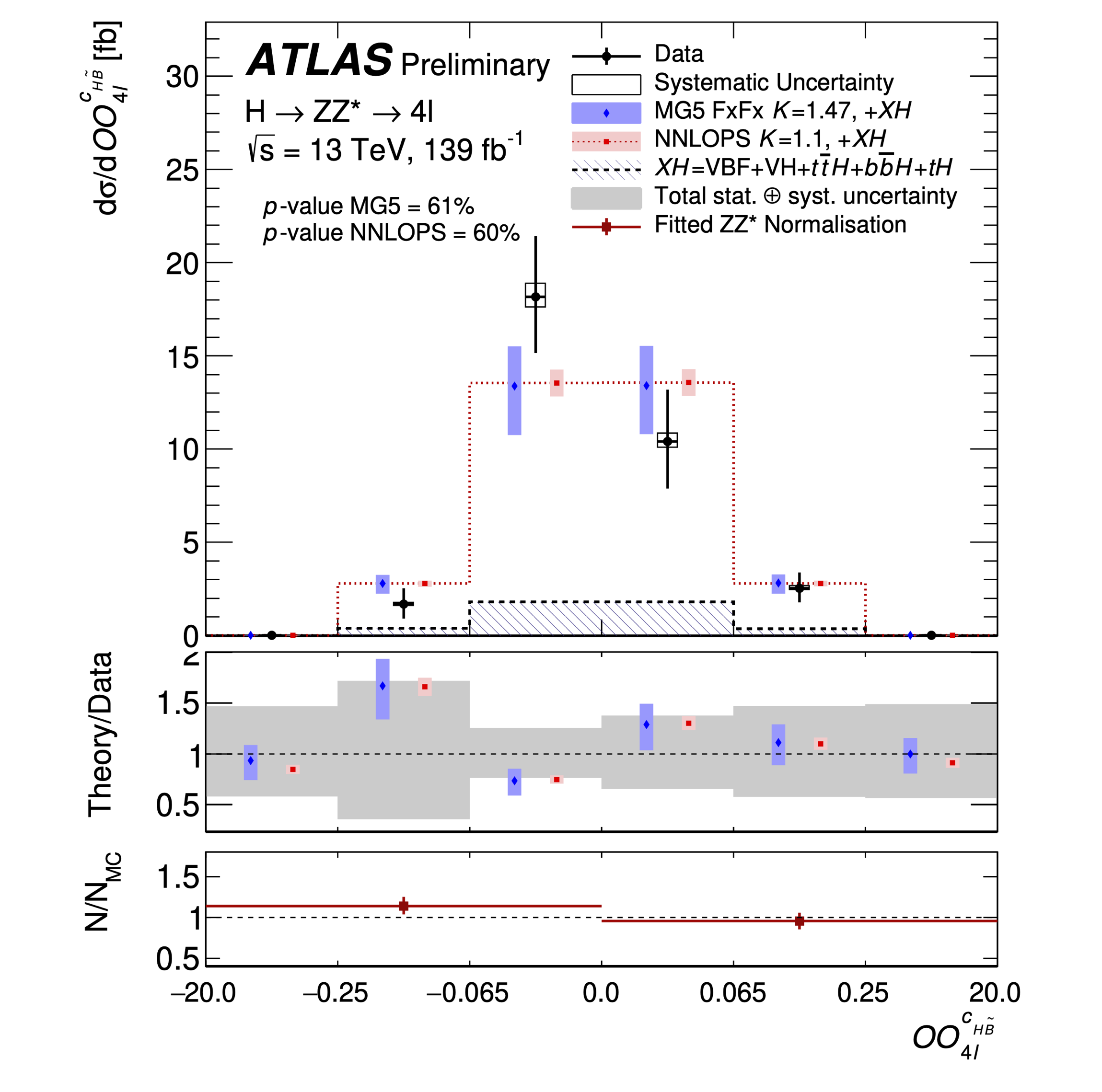Tags:

# Probing fundamental symmetries of nature with the Higgs boson

31 March 2023 | By

Where did all the antimatter go? After the Big Bang, matter and antimatter should have been created in equal amounts. Why we live in a Universe of matter, with very little antimatter, remains a mystery. The excess of matter could be explained by the violation of charge-parity (CP) symmetry – which essentially means that certain processes that involve particles and their antiparticles behave differently.

However, the CP-violating processes that have been observed so far are too small to explain matter-antimatter asymmetry. New sources of CP violation must be out there – and might be hiding in interactions involving the Higgs boson. In the Standard Model, Higgs-boson interactions with other particles conserve CP symmetry (“CP-even”). If researchers find signs of CP violation in these interactions (“CP-odd” components), these could be a clue to one of the Universe’s oldest mysteries.

In the new results presented at the Moriond conferences, the ATLAS Collaboration tested the CP invariance of Higgs-boson interactions with vector bosons (W or Z bosons). They studied Higgs-boson decays into two vector bosons, each of which transform into a lepton-anti-lepton pair (electron-positron or muon-anti-muon). Researchers also studied interactions where two vector bosons combine to produce a Higgs boson (vector boson fusion, VBF). In this case, one quark and one anti-quark are produced together with the Higgs boson, creating ‘jets’ of particles in the ATLAS detector.

These interactions are ideal testing grounds for CP violation. When CP symmetry is preserved, the pattern of behaviour of the detected jets and leptons should be the same when exchanging particles with their antiparticles and flipping their directions of flight. However, if CP symmetry is violated, then particles and antiparticles behave differently.

### The ATLAS Collaboration tested Higgs-boson interactions with vector bosons, looking for signs of charge-parity (CP) symmetry violation.

ATLAS scientists summarise all the information about the detected particles in a single number: the optimal observable. A special feature of this observable is that its value measured for antiparticles should be equal but opposite to that of the particles. As shown in Figure 1, the shape of the optimal observable distribution becomes asymmetric in the presence of CP violation. If the process preserves CP symmetry, the mean value of the optimal observable in the data should be zero.

As the name suggests, these observables are optimised to maximise the separation between different possible outcomes of a process. In this analysis, researchers calculate these numbers from the theoretical probability of CP-even (Standard Model) and CP-odd (new-physics phenomena) reactions occurring. But how can scientists foresee new-physics processes? A theoretical extension of the Standard Model called Effective Field Theory (EFT) provides a simple way of expressing how new particles too heavy to be produced at LHC could indirectly affect ATLAS measurements through quantum physical effects.

In ATLAS’ new analysis, physicists used the observed distributions to directly constrain CP-odd couplings (see Figure 2). The "limits" they set on the couplings are the possible largest values that are still compatible with the data. These were obtained in three different ways: looking only at VBF production, only at the four-lepton decays, and by combining both. In each case, collision events were categorised in order to maximise the analysis sensitivity. For example, in the VBF-only study, researchers used an artificial-intelligence algorithm to discriminate VBF events from the other main Higgs-boson production mode (gluon-gluon fusion). When looking solely at four-lepton decays, researchers focused only on events within the Higgs-boson mass range (115 GeV to 130 GeV).

Researchers also measured how often each value of optimal observable occurred in the data – after correcting for any experimental effects – to provide differential cross section measurements (see Figure 3). This measurement enabled scientists to compare the experimental data with theoretical predictions in a model-independent way and test the validity of the underlying theoretical assumptions. This is the first time that a measurement in the four-lepton final state has allowed physicists to detect signs of CP violation in a model-independent way, without strongly relying on other aspects of the Standard-Model prediction aside from CP-symmetry.

All the results look compatible with the Standard Model expectation – another important confirmation of the current theory of nature! However, this is just the first step. Small CP-violating signals remain compatible with the data, and the ATLAS Collaboration is already collecting new collision data at unprecedented energies that will allow to increase the precision of these measurements – further homing in on the nature of the Higgs boson.Figure 2: Summary of results obtained with this analysis on different CP-odd couplings, representing various ways CP-violation could be realised according to the EFT approach. The points show the values of each coupling measured from data and the bars correspond to the limits of those couplings within 68% of confidence level (CL). The observed results for the CP-odd couplings are all consistent with zero, in agreement with the Standard Model. (Image: ATLAS Collaboration/CERN)Figure 3: The measured probability of recording different values of the optimal observable. Black markers show measured probabilities, expressed as a “scattering cross section”, which relates frequency of observation to the LHC-beam properties. The red dashed line shows the predicted Standard-Model distribution. Two calculations of this prediction (blue and red markers) are compared, and found to yield comparable results. Lower panel gives the ratio of the prediction to the measured value. The shaded grey band indicates the uncertainty on the measurement. (Image: ATLAS Collaboration/CERN)